# Particle Size Analysis of Pigments Using Laser Diffraction

Pigments and paints play a key role as industrial materials. The properties of a given pigment/paint system are determined largely by the particle size distribution. Particle size determines the tinctorial strength or the depth of color, furthermore, it may also be an important physical parameter of the pigment system itself.

For example, in printing inks, it is essential that the ink particles are not larger than the nozzle delivery system that supplies the ink. The ability of a given pigment to absorb light (tinctorial strength) increases with decreasing particle diameter and increased surface area, until it reaches a point when the particles become translucent to the incident light. This single factor alone makes the measurement of particle size critical to the performance for many pigment applications.

## Sizing Pigments Using Laser Diffraction Analysis

A wide range of technologies have been employed to measure the particle size distributions of pigment systems. Among these, laser diffraction has increasingly become the most commonly employed. The ease of use coupled with a short analysis time, mostly less than one minute, has made laser diffraction the primary method by many companies for process control.

It is important to first understand why laser diffraction particle size analyzers have difficulties sizing submicron materials. When illuminated by a laser beam, large particles scatter light strongly at small angles and with readily detectable maxima and minima in the scattering pattern. This means that detectors placed at small angles, relative to the optical path and with sufficient angular resolution, can detect the fine detail in the scattering pattern.

It is the precise measurement of these maxima and minima that allows the determination of the mean size, as well as size distribution, of the analyzed material. Conversely, small particles scatter light weakly and without any discernible maxima and minima until high angles of measurement are reached. As can be seen in Figure 1, once there are particles below 1 μm, many difficulties in the measurement are encountered due to weak scattering signals and smooth angular patterns. There is no distinguishable feature in the scattering pattern that can be characterized to determine the actual particle size.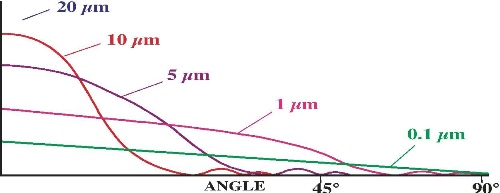Figure 1. The weak scattering signal from particles below 1 μm in size present difficulties in measuring the maxima or minima at high angles. Image credit: Beckman Coulter

By using a shorter wavelength, the ratio between particle size and light wavelength is increased thus increasing the ability to measure smaller particles. Beckman Coulter, has discovered a novel technique for enhancing submicron sizing in standard laser diffraction systems. This involved the utilization of polarization effects of scattered light of varies wavelengths apart from the main diffraction laser source in a broad angular range. The technique is called PIDS, which stands for Polarization Intensity Differential Scattering.

## PIDS

The technology used in PIDS is simple and has been derived from the well-established and understood Mie theory of light scattering. PIDS relies upon the transverse nature of light. It comprises a magnetic vector and an electric vector perpendicular to each other. If, for example, the electric vector is “up and down,” the light is said to be vertically polarized. When a sample with light of a given wavelength and polarization is illuminated, the electric field establishes a dipole. The oscillations of the electrons in this dipole will be in the same plane of polarization as the propagated light source.

The oscillating dipoles in the particles disperse light in all directions except that of the oscillation. PIDS takes advantage of this phenomenon. Three wavelengths (450 nm, 600 nm and 900 nm in the Beckman Coulter LS 230 and LS 13 320) sequentially illuminate the sample, first with vertically and then with horizontally polarized light. The scattered or reradiated light from the sample is then determined over a range of angles. The differences between the vertically and the horizontally polarized light for each wavelength are analyzed giving information about the particle size distribution of the sample.

It is essential that the differences between the vertically and the horizontally polarized signals are being measured, and not simply the values at a given polarization. The intensity vs. scattering angle information from the PIDS signals is then combined with the intensity vs. scattering angle data from the primary laser of the instrument to give a continuous size distribution from submicron to millimeter (0.04 μm to 2,000 μm in Beckman Coulter LS 230 and LS 13 320).

## The Problem with Pigments

Pigments offer a unique problem not encountered with most materials that are measured using laser diffraction instruments. For sizing colored pigment samples precisely, both the real refractive index of the material and its imaginary component must be known. While the real refractive index is a value that can be obtained by a number of analysts, the determination of the imaginary component is not trivial. It is the degree of absorbance that is shown by the sample at a given wavelength. White or transparent materials show no absorbance.

For pigments, they are colored lies with the fact that they absorb certain wavelengths preferentially. For example, when a blue pigment with an absorbance maximum at 630 nm interacts with a helium neon laser (wavelength 633 nm), which is the choice of a number of manufacturers for their primary laser light source, the pigment essentially behaves as a black body. This must be considered when calculating the particle size distribution, particularly if the particles are small. It is easy to see that the quantification of the imaginary component of the complex refractive index is very important for the accurate determination of pigment particulate systems.

## Determining the Imaginary Component

Determining the imaginary component of a pigment can be performed using a UV/Vis spectrophotometer, which measures the relative absorbency of a material per given wavelength. To permorm spectrophotometer measurements,  a liquid that dissolves the pigment particles into molecules has to be utilised.The light attenuation, when passing through the sample, is due to both absorption and scattering. One needs to minimize scattering in order to measure absorption correctly. For colored materials, one needs to obtain the imaginary component of complex refractive index for each wavelength and use them selectively to calculate a complete Mie theory optical model for a given sample.

During analysis of pigments, it is also beneficial to utilize other sources of information to initially check or confirm the results obtained. Once the correlating information has been proven the be suitable for a given model of a particular sample it can then be used with confidence for that material.

The best sources of correlating information are photomicrographs. These can be images from optical microscopes or electron microscopes. This approach is particularly important for the detection of small amounts of oversized materials. This can be a common problem with many pigment systems due to the type of size reduction or milling techniques utilized. Ball mills can give rise to small amounts of oversized materials that may remain undetected by laser diffraction, especially if the imaginary component of the refractive index is not taken into account. The suitability of an optical model for a given pigment is best determined if one tracks a milling process over time. If the correct refractive index values have been used to create the optical model, one should obtain a constant reduction of the mean size.

Figures 2-4 show examples of using correct imaginary refractive index when sizing pigment particles. The data in Figure 3 were the same as the ones in Figure 2 but analyzed using the imaginary values for a blue pigment. Note the narrow distribution and the lack of the population of oversized particles.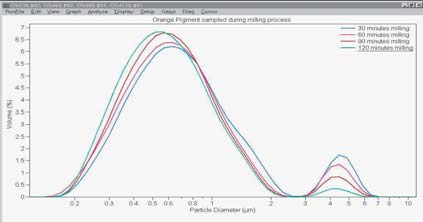Figure 2. Size distributions of an orange pigment progressively milled over a two-hour period. Maximum UV/Vis absorbance was shown at approximately 450 nm. Image credit: Beckman Coulter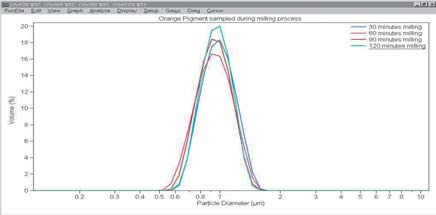Figure 3. Size distributions from the same data in Figure 2 but with a wrong imaginary refractive index value. Image credit: Beckman Coulter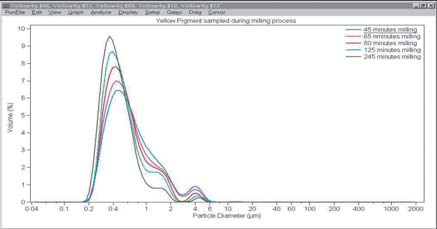Figure 4. Yellow pigment, milled during a four-hour period, yields a meaningful distribution by using imaginary values of the refractive index that were determined by UV/Vis spectroscopy. The distribution may also be corroborated by using other methods. Image credit: Beckman Coulter

## The Question of Shape

All laser diffraction instruments have a major drawback: they make no allowance for the shape of the materials under test, regardless of the size of the particles. The reasons for this lie with the underlying assumptions, used in calculating size distributions from the raw data generated during the analysis. The mathematical models used to calculate distributions are based on scattering of light by a sphere.

Any reported distribution is, in effect, an equivalent spherical distribution of the material being studied. In most instances this is quite adequate since most particles approximate to a spherical system adequately enough.

One example where the spherical shape assumption is not accurate is that of a mono-disperse, spheroidal material of submicron hematite spindles that are 46.9 nm in width and 130.8 nm in length, giving them an approximate aspect ratio of 3:1, as determined by scanning electron microscopy (SEM) (Fig. 5).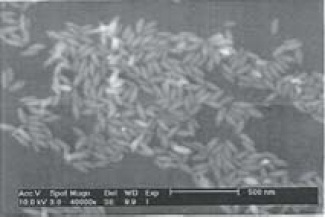Figure 5. The SEM photomicrograph shown is a representative sample of hematite spindles. Image credit: Beckman Coulter

The optical properties of the hematite spindles have been measured using UV/Vis spectroscopy for the imaginary component and from UV/Vis spectroscopic ellipsometry for the real component of the refractive index. The reported value for the mean size using the Beckman Coulter LS 13 320 is 78 nm (Fig. 6) which is well within the range of what one would expect, given the random motion of the particles as they traverse through the illuminated beam during measurement.

## Summary

Enhanced multi-wavelength laser diffraction can be employed successfully to size particulate pigment samples. The refractive index can be determined using several means. It is also worthwhile considering using other techniques to initially corroborate the results obtained.This information has been sourced, reviewed and adapted from materials provided by Beckman Coulter, Inc. - Particle Characterization.